### Home > CALC > Chapter 1 > Lesson 1.2.3 > Problem1-56

1-56.
1. Wei Kit loves shortcuts! When calculating with fractional exponents, he looks for a way to avoid using his calculator. For example, he found out that 82/3 = 4 by using the method below: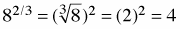Use Wei Kit's method to evaluate the following expressions: Homework Help ✎

1. 1003/2

2. 274/3

3. 163/4

4. 94/2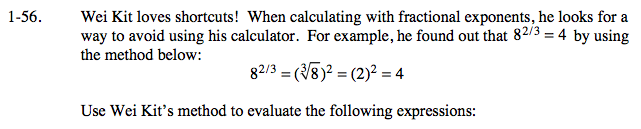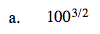To avoid dealing with large numbers, find the square root of 100 first.

$\text{Afterall, } 100^{ \frac{1}{2} }\text{ is equivalent to }\sqrt{100}.$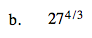Step 1: What is the cube-root of 27?

Step 2: Raise the cube-root of 27 to the 4th-power.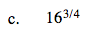What is the fourth-root of 16?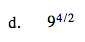$\text{Reduce \frac{4}{2}\ before evaluating.}$